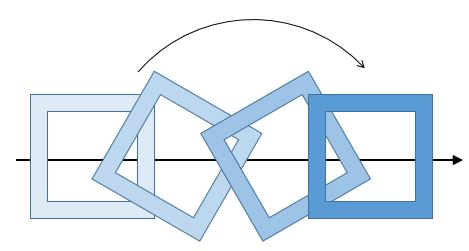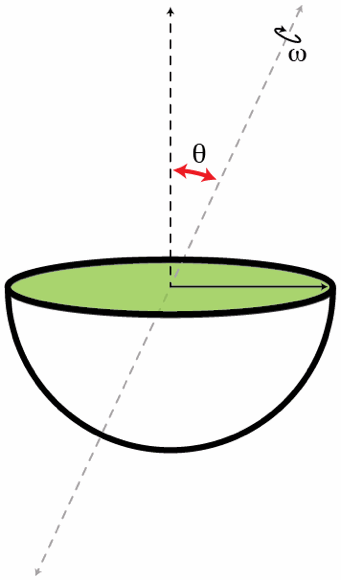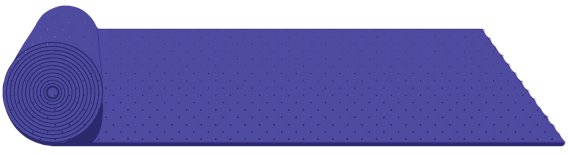Classical Mechanics

Rotational Kinetic Energy: Level 3-4 Challenges

A wheel is rotating at an angular speed of 20 rad/s. It is stopped to rest by applying a constant torque in 4 seconds. If the moment of inertia of the wheel about its axis is 0.20 kg m$^2$, then what is the magnitude of the work done by the torque in first two seconds?A frustrated painter grabs a wooden frame and throws it through his studio. As the frame flies through the air, it rotates about its central perpendicular axis. (See drawing for an idea of the shape and motion.)

The painter's wife, being a student of physics, observes that whenever a corner of the frame is at the bottom, it is instantaneously at rest.

What percentage of the frame's kinetic energy is rotational kinetic energy?

Assumptions and information

The frame and the hole in it are square and concentric. The side of the square hole is 90% of the side of the frame. The mass is uniformly distributed in the frame.

For a uniform square of side $a$ with a hole of side $b$, the moment of inertia about the perpendicular axis through the center is $I = \frac 1 6 m (a^2 + b^2.)$

Ignore the effect of gravity on the path of the frame; assume it is moving in a straight line.A solid hemisphere of radius $R=0.5~\mbox{m}$ and mass $M=1~\mbox{kg}$ rotates with angular speed $\omega=10~\text{rad/s}$ about an axis passing through its center O and making and angle $\theta=60^{\circ}$ with the vertical (see the figure below). Determine the kinetic energy in Joules of the hemisphere.A carpet is rolled into the form of a cylinder (by rolling it along the length) of radius $\frac{27}{140}\text{ m}$ and it is kept on a rough horizontal floor. The carpet is given a negligible (gentle) push and it starts to unroll without slipping. Calculate the horizontal velocity in $\text{m}/\text{s}$ of the axis of the cylindrical part of the carpet when its radius becomes $\frac{27}{280}$.

Note: Approximate $g$ to be $10 \text{ m}/\text{s}^2.$

An ice skater is standing with his arms wide, spinning on the tip of one skate. By pulling his arms in, his moment of inertia is reduced to 60% of its original value.

If $K$ is the original rotational kinetic energy of the skater and $W$ is the work done in pulling in his arms, what is the ratio $W:K$?

×